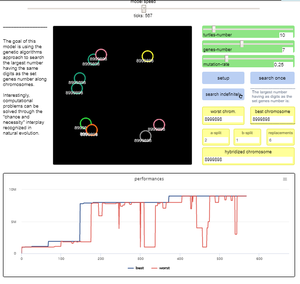# MGA - Minimal Genetic Algorithm (version 1.1.0)

Genetic algorithms try to solve a computational problem following some principles of organic evolution. This model has educational purposes; it can give us an answer to the simple arithmetic problem on how to find the highest natural number composed by a given number of digits. We approach the task using a genetic algorithm, where the candidate solutions to the problem are represented by agents, that in logo programming environment are usually known as “turtles”.#### Release Notes

See the info-tab attached to the code file